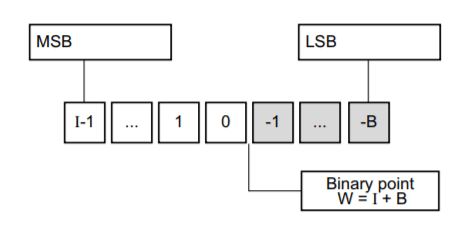# MicroZed Chronicles: Tips and Tricks When Working with HLS — Part Two

### MicroZed Chronicles: Tips and Tricks When Working with HLS — Part Two

In last week’s blog we looked at how we could use Vivado High Level Synthesis to implement and optimize a complex industrial equation.Fixed point representation in HLS /FPGA

Of course the implementation used floating point representation of numbers, and therefore required considerable resources — significantly more than if we had implemented the algorithm using a fixed point representation.

In this blog, we are going to examine how we work with fixed point representations and our options for doing so.

When we work with fixed point numbers, we have to quantize the floating point number into one that can be represented in a fixed point system. Doing so may result in a loss of accuracy, which is why is is common to use vectors of arbitrary length to obtain sufficient accuracy when we implement HDL-based fixed point solutions.

The normal way of representing the split between integer, fractional bits in a vector with a fixed-point number is x,y where x represents the number of integer bits and y the number of fractional bits. For example, 8,8 represents 8 integer bits and 8 fractional bits while 16,0 represents 16 integers and 0 fractional. This format is often called Q format, sometimes given as Qm.n where m represents the number of integer bits and n represents the number of fractional bits.

For example, Q8,8 is capable of representing an unsigned number of between 0.0 and 255.9906375

When working with HLS, in order to be able to implement fixed point numbers accurately, we need to be able create vectors which are not limited to 8,16, 32 or 64 bits.

This is where the Vivado HLS arbitrary precision libraries come into play, and we have several options depending upon the language we are working with as to which one we use.

For all HLS supported languages — C, C++ and System C — there is an arbitrary precision library available, which allows us to create signed or unsigned integers sized up to 1024 bits (System C is limited to 512).

If we desire, we can perform mathematical operations using these arbitrary precision libraries; however to do so we need to take into account the following:

• Conversion of floating point to fixed point number representation
• Impact of rounding / truncation using a fixed point system
• Alignment of the decimal points in fixed point number representations for some mathematical operations.
• Correctly sizing the variable which stores the result of the operation

But for mathematical operations, there is a better alternative if we are working with C++ or System C. This is making use of the Arbitrary Precision Fixed Point library.

Using fixed point data types enables us to not only obtain simulations which are identical to the resulting hardware implementation, but also lets us control the following:

• Width of the integer (W)
• Number of integer bits (I)
• Quantization mode (Q) — select one of seven quantization schemes e.g. convergent rounding or truncation, etc.
• Overflow behavior (O) — define how the variable reacts when the result overflows e.g. saturate, wrap around, etc.
• Saturation bit (N) — used in support of the overflow wrap modes.

Obviously if we are mathematical operations, the fixed point data types are much easier easier to work with than the arbitrary precision. Especially as they provide the:

• Decimal point alignment for mathematical operations is handled automatically.
• Easy conversion of number to a fixed point representation.

Let’s take a look at a simple example, like we did last week. For this example we will implement a simple moving average filter. These can be used in noisy environments to filter out random noise.

A moving average filter simply sums up a number of inputs values and determines the average of those inputs. For this example, 10 input values each of 10 bits with 8 integer and 2 fractional bits will be averaged.Fixed point code when using a

Again we can use the FIFO interface type for the array input and pipeline the loop. This time, as we are working with fixed point representations and not floating point representations, we can achieve an initiation interval of 1 which really helps out throughput.

The tips and tricks presented in the last two blogs can really help you leverage HLS to create modules that meet challenging performance requirements.

You can find the files associated with this project here:

ATaylorCEngFIET/MicroZed-Chronicles

See previous projects here.

More on on Xilinx using FPGA development weekly at MicroZed Chronicles.MicroZed Chronicles: Tips and Tricks When Working with HLS — Part Two was originally published in Hackster Blog on Medium, where people are continuing the conversation by highlighting and responding to this story.

Original article: MicroZed Chronicles: Tips and Tricks When Working with HLS — Part Two